# Mongo DB Atlas and R

At One Earth Future Research our mission is to utilize rigorous research methodologies in an effort to provide empirical assessment of the root-causes of political violence and the efficacy of governance and development programs. I will be starting a semi-weekly blog that highlights some of our work in both the conflict trends and forecasting tracks. Our collective goal is to utilize data to explore the mechanisms of conflict and the long-term shifts in conflict related phenomena (conflict trends) and to understand and predict short term outcomes in conflict and political violence (forecasting). Our organization utilizes field work and in-depth interviews alongside state of the art technical approaches found in inferential statistics and machine learning/artificial intelligence.

### Setup and Data

As we went about the process of creating a centralized data repository for all organizational data, we stumbled upon MongoDB’s Atlas platform. Atlas utilizes the MongoDB document system and is accessible from the cloud (AWS, Google Cloud, or Azure). This blog provides some demonstration code for utilizing Atlas in the R programming environment with our soon-to-be released election violence data.

#### Our data

We have a data set of all major national elections from 1975 to 2018 that is based on original data gathering and the NELDA dataset put together by Hyde and Marinov1 and our own REIGN dataset2. For this demonstration, we will simply use the name ev_df for our new election violence data for the time being.

Let’s quickly inspect our data before moving it into Atlas.

summary(ev_df)
##       year                          country            dates
##  Min.   :1975   Ecuador                 :  35   10/25/2015:   8
##  1st Qu.:1990   United States of America:  33   10/24/1999:   6
##  Median :2000   Iran                    :  32   10/23/2011:   5
##  Mean   :1999   France                  :  31   10/31/1999:   5
##  3rd Qu.:2009   Egypt                   :  29   11/28/2010:   5
##  Max.   :2018   Guatemala               :  29   11/28/2011:   5
##                 (Other)                 :2074   (Other)   :2229
##  elecViolence l.elecViolence  anticipation     regimetenure
##  vio  : 617   0:1645         Min.   :0.0000   Min.   :  1.00
##  peace:1623   1: 618         1st Qu.:0.0000   1st Qu.: 27.00
##  NA's :  23                  Median :1.0000   Median : 56.00
##                              Mean   :0.6879   Mean   : 83.39
##                              3rd Qu.:1.0000   3rd Qu.:109.00
##                              Max.   :1.0000   Max.   :589.00
##
##   lastelection       pcgdp              growth            logIMR
##  Min.   :0.000   Min.   :     0.0   Min.   :0.09887   Min.   :0.6419
##  1st Qu.:0.000   1st Qu.:   709.6   1st Qu.:0.99141   1st Qu.:2.3842
##  Median :0.000   Median :  2143.7   Median :1.05674   Median :3.3464
##  Mean   :1.068   Mean   :  7269.8   Mean   :1.06457   Mean   :3.2260
##  3rd Qu.:2.441   3rd Qu.:  7933.4   3rd Qu.:1.12739   3rd Qu.:4.1558
##  Max.   :8.098   Max.   :102573.7   Max.   :4.09959   Max.   :5.1728
##
##      lnpop2         logpredict             SPI               lexconst
##  Min.   : 31.48   Min.   :0.0000221   Min.   :-2.561111   Min.   :1.000
##  1st Qu.: 69.81   1st Qu.:0.0006450   1st Qu.:-0.428677   1st Qu.:3.000
##  Median : 84.67   Median :0.0015977   Median : 0.023829   Median :5.000
##  Mean   : 88.17   Mean   :0.0038759   Mean   :-0.001438   Mean   :4.803
##  3rd Qu.:105.59   3rd Qu.:0.0037408   3rd Qu.: 0.428446   3rd Qu.:7.000
##  Max.   :197.67   Max.   :0.1414839   Max.   : 2.682971   Max.   :7.000
##
##     lpolity2          lpolcomp
##  Min.   :-10.000   Min.   : 1.000
##  1st Qu.: -4.000   1st Qu.: 3.000
##  Median :  6.000   Median : 7.000
##  Mean   :  3.186   Mean   : 6.603
##  3rd Qu.:  9.000   3rd Qu.: 9.000
##  Max.   : 10.000   Max.   :10.000
## 

The data contains 17 variables and captures 2263 unique elections across the 1975 - 2018 time period.3

As of right now, we have no data housed on our Atlas cluster called OEFdata. To send data to our Atlas cluster, we can use the mongolite package.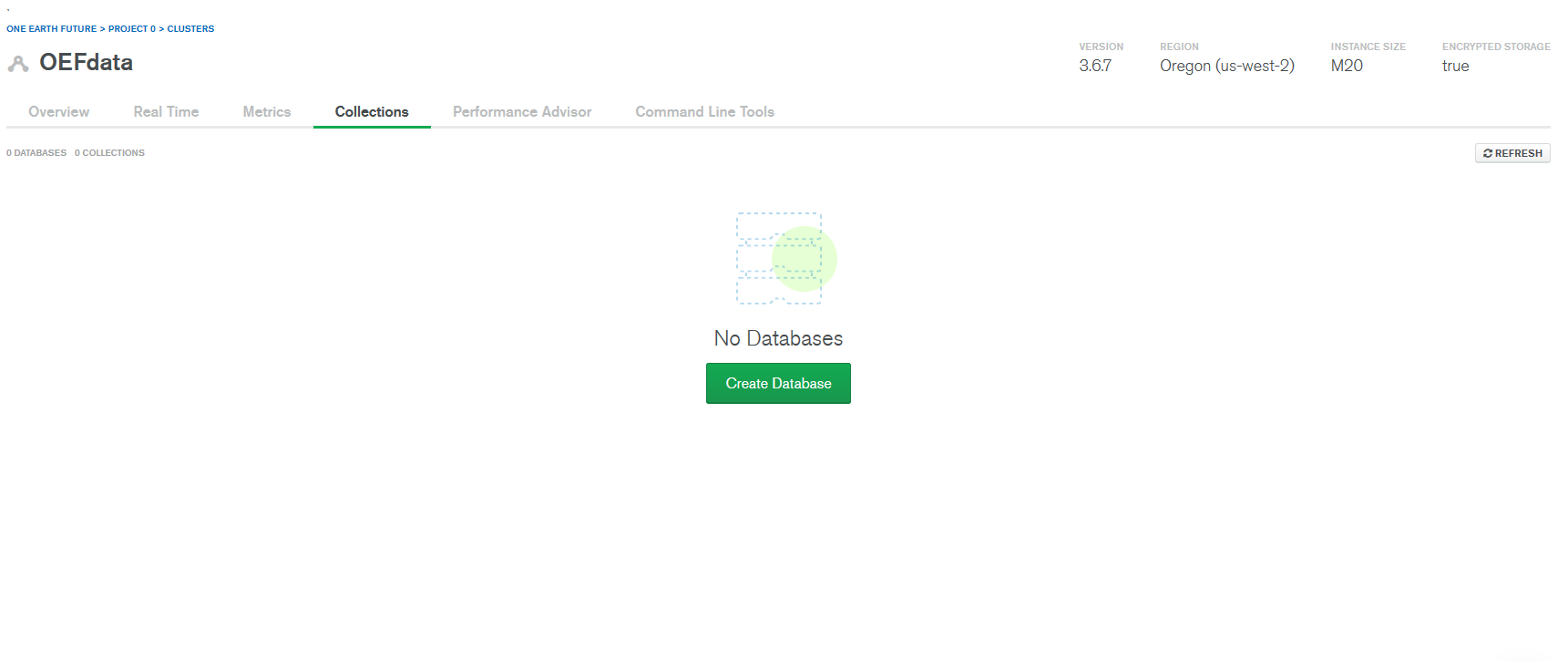Empty atlas database

library(mongolite)

#make connection object that specifies new database and collection (dataset)
mongo1 <- mongo(collection = "EV_Forecast Data (1975 - 2018)", db = "OEFR",
url = url_path,
verbose = TRUE)

#show commands for connection
mongo1
## <Mongo collection> 'EV_Forecast Data (1975 - 2018)'
##  $aggregate(pipeline = "{}", options = "{\"allowDiskUse\":true}", handler = NULL, pagesize = 1000, iterate = FALSE) ##$count(query = "{}")
##  $disconnect(gc = TRUE) ##$distinct(key, query = "{}")
##  $drop() ##$export(con = stdout(), bson = FALSE, query = "{}", fields = "{}", sort = "{\"_id\":1}")
##  $find(query = "{}", fields = "{\"_id\":0}", sort = "{}", skip = 0, limit = 0, handler = NULL, pagesize = 1000) ##$import(con, bson = FALSE)
##  $index(add = NULL, remove = NULL) ##$info()
##  $insert(data, pagesize = 1000, stop_on_error = TRUE, ...) ##$iterate(query = "{}", fields = "{\"_id\":0}", sort = "{}", skip = 0, limit = 0)
##  $mapreduce(map, reduce, query = "{}", sort = "{}", limit = 0, out = NULL, scope = NULL) ##$remove(query, just_one = FALSE)
##  $rename(name, db = NULL) ##$replace(query, update = "{}", upsert = FALSE)
##  $run(command = "{\"ping\": 1}", simplify = TRUE) ##$update(query, update = "{\"$set\":{}}", filters = NULL, upsert = FALSE, multiple = FALSE) Use the$insert command to store the data in your Atlas collection.

mongo1$drop() mongo1$insert(ev_df)
##
Processed 1000 rows...
Processed 2000 rows...
Complete! Processed total of 2263 rows.
## List of 5
##  $nInserted : num 2263 ##$ nMatched   : num 0
##  $nRemoved : num 0 ##$ nUpserted  : num 0
##  $writeErrors: list()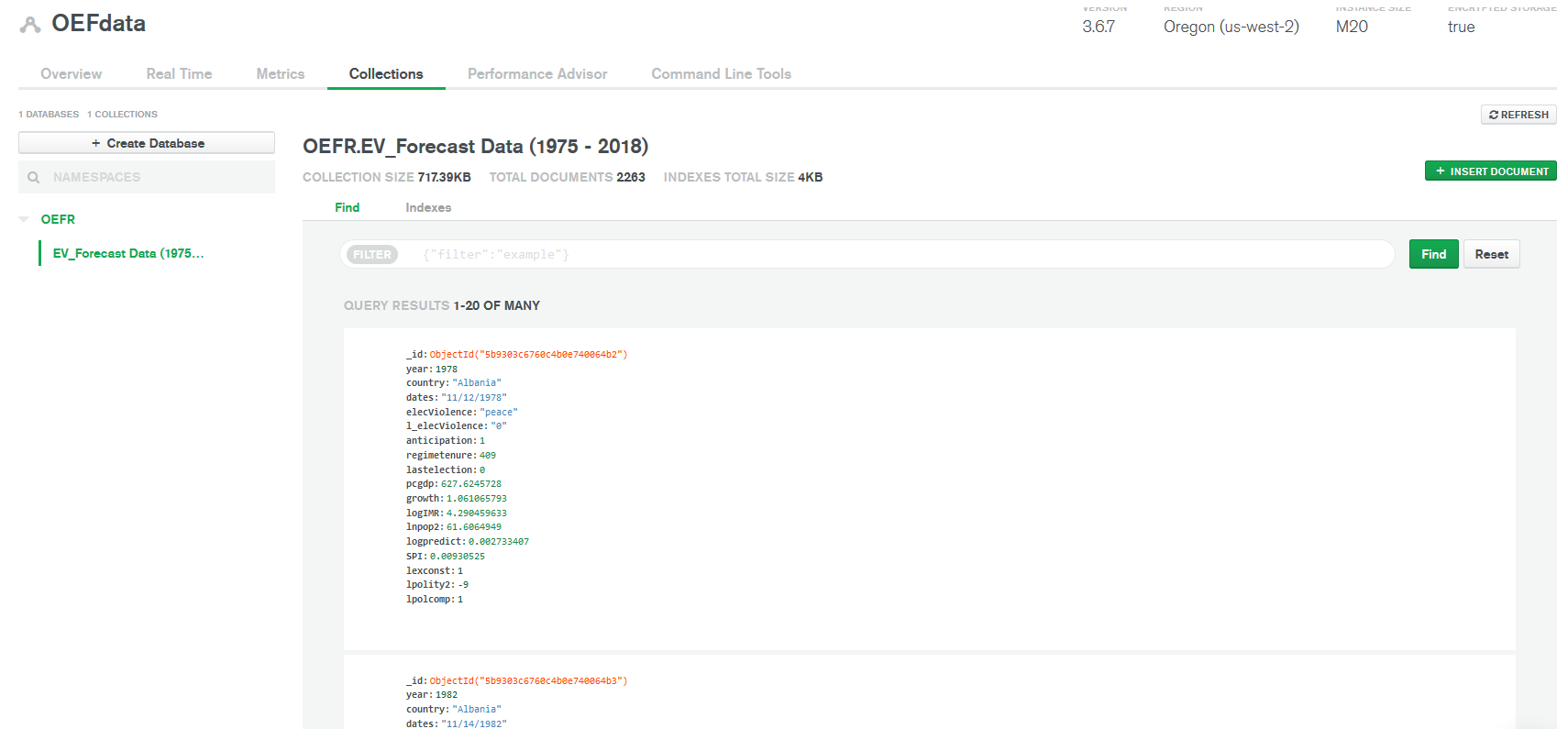Our election violence data (collection) is now housed in our OEFR database #### Analysis commands and pulling data into R. Now our original R dataframe is hosted in our Atlas cluster in the MongoDB document format and we can start to analyze and manipulate data that is already stored in our cluster. #count number of documents (rows) mongo1$count()
##  2263
#import data frame back into R

ev_df2 <- mongo1$find() ## Found 1000 records... Found 2000 records... Found 2263 records... Imported 2263 records. Simplifying into dataframe... str(ev_df2) ## 'data.frame': 2263 obs. of 17 variables: ##$ year          : int  1978 1982 1987 1991 1991 1992 1992 1996 1996 1997 ...
##  $country : chr "Albania" "Albania" "Albania" "Albania" ... ##$ dates         : chr  "11/12/1978" "11/14/1982" "2/1/1987" "3/31/1991" ...
##  $elecViolence : chr "peace" "peace" "peace" "vio" ... ##$ l_elecViolence: chr  "0" "0" "0" "0" ...
##  $anticipation : num 1 1 1 1 1 1 1 1 1 1 ... ##$ regimetenure  : num  409 457 23 72 73 84 84 50 51 63 ...
##  $lastelection : num 0 0 0 0 0 0 0 0 0 0 ... ##$ pcgdp         : num  628 798 807 410 410 ...
##  $growth : num 1.061 1.008 0.973 0.611 0.611 ... ##$ logIMR        : num  4.29 4.03 3.71 3.52 3.52 ...
##  $lnpop2 : num 61.6 62.9 64.7 65.6 65.6 ... ##$ logpredict    : num  0.00273 0.00222 0.00236 0.00302 0.00329 ...
##  $SPI : num 0.00931 0.25154 -0.39167 -1.55961 -1.2355 ... ##$ lexconst      : int  1 1 1 3 3 3 3 5 5 5 ...
##  $lpolity2 : int -9 -9 -9 1 1 1 1 5 5 0 ... ##$ lpolcomp      : int  1 1 1 6 6 6 6 6 6 6 ...

The find function can also be used for making conditional queries from our Atlas cluster, and is especially useful for big data that may not play well with your local R instance. Lets demonstrate some ways to query/subset from our election violence data.

#use the query argument to subset by rows
#lets get all observations that experienced election violence

ev_df2 <- mongo1$find(query = '{"elecViolence":"vio"}') ## Found 617 records... Imported 617 records. Simplifying into dataframe... #inspect our subsetted data frame nrow(ev_df2) ##  617 #use the fields argument to subset by columns #lets query the above data, but only getting country and date information ev_df2 <- mongo1$find(query = '{"elecViolence":"vio"}',
fields = '{"country": true, "dates": true}')
##
Found 617 records...
Imported 617 records. Simplifying into dataframe...
#inspect data frame variables

summary(ev_df2)
##      _id              country             dates
##  Length:617         Length:617         Length:617
##  Class :character   Class :character   Class :character
##  Mode  :character   Mode  :character   Mode  :character

Now we can create a new cluster collection to host our subsetted data

#now lets upload our new subset into it's own Atlas collection
#make new connection within the OEFR database

mongo2 <- mongo(collection = "Election Violence Events (Country, Dates)", db = "OEFR",
url = url_path,
verbose = TRUE)

mongo2$insert(ev_df2) ## Complete! Processed total of 617 rows. ## List of 5 ##$ nInserted  : num 617
##  $nMatched : num 0 ##$ nRemoved   : num 0
##  $nUpserted : num 0 ##$ writeErrors: list()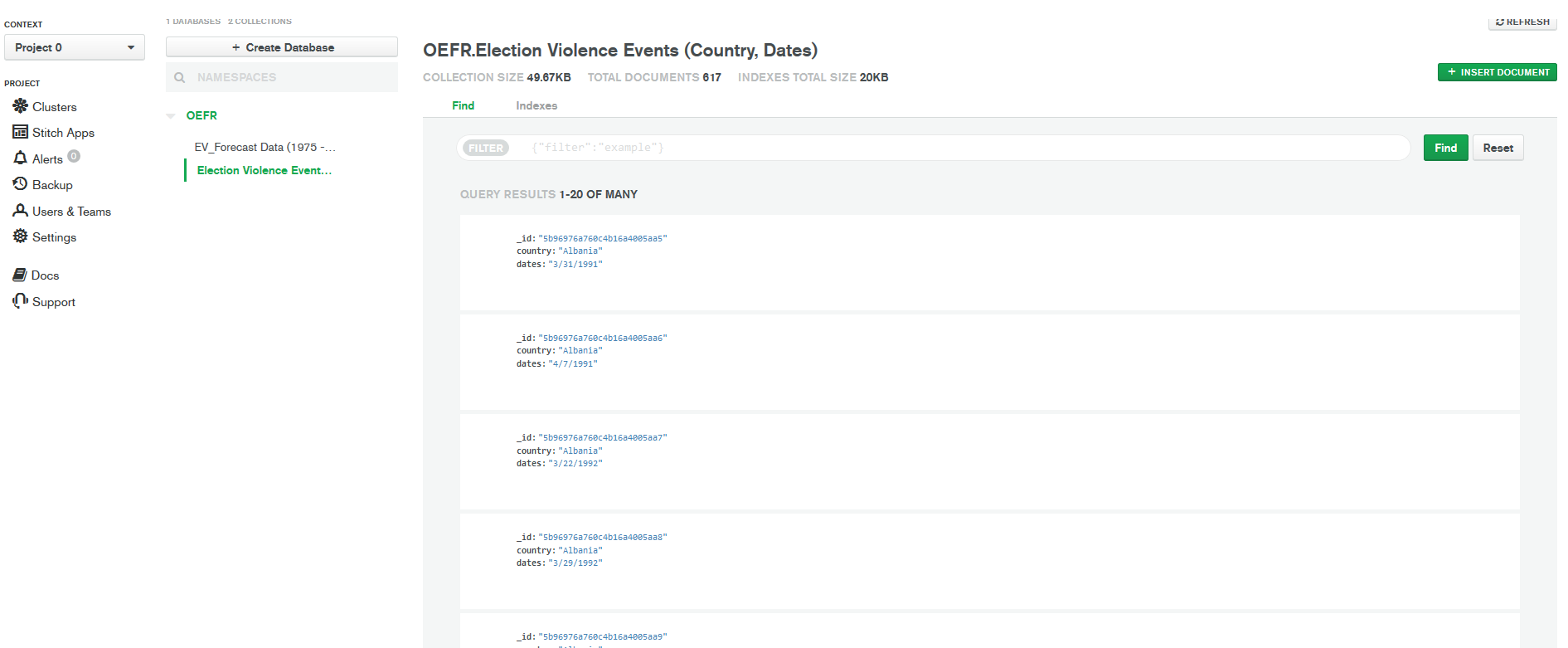We now have a new collection of just election violence events!

If you need to delete a a collection (data frame) from your Atlas cluster, you can use the following.

#drop and delete collection tab in Atlas

mongo2$drop() #drop data frame, but keep collection tab in Atlas mongo2$drop{'{}'}

Finally, we can query aggregate statistics from our Atlas cluster using MongoDB’s pipeline syntax. Aggregating at the group level is useful for getting more managable data sets when dealing with big data. Lets demonstrate this capability using our election violence data!

#Lets get the count of country elections and the average infant mortality rate and SPI (precipitation) by country

agg_stats <- mongo1$aggregate('[{"$group":{"_id":"$country", "election events": {"$sum":1},
"average IMF":{"$avg":"$logIMR"}, "average SPI":{"$avg":"$SPI"}}}]'
)
##
Found 152 records...
Imported 152 records. Simplifying into dataframe...
#show first 25 rows of country aggregated data
head(agg_stats, n = 25)
##                         _id election events average IMF average SPI
## 1                  Zimbabwe              19    4.043437  0.33245826
## 2                 Venezuela              21    3.006033  0.20093259
## 3                Uzbekistan              12    3.817488 -0.02127261
## 4  United States of America              33    2.115447 -0.03168326
## 5            United Kingdom              10    1.810915 -0.07704831
## 6                   Ukraine              19    2.579029  0.21402413
## 7                  Thailand              22    3.090824  0.24227424
## 8                    Sweden              13    1.465030 -0.17207021
## 9                 Swaziland               6    4.205235 -0.12403276
## 10                  Surinam               2    3.020008 -0.92425866
## 11                    Sudan              12    4.218346 -0.11023233
## 12                    Spain              12    1.880806 -0.24571050
## 13             South Africa               9    4.058460  0.04487938
## 14                  Somalia               4    4.662905 -0.62259620
## 15          Solomon Islands               2    3.233632  0.18578571
## 16                    Syria              17    3.159167 -0.26130308
## 17                 Slovakia              13    2.104528  0.06276020
## 18                Singapore              13    1.447405  0.43001852
## 19      Serbia (Yugoslavia)               3    3.039749 -0.64691414
## 20                  Senegal              18    4.168331 -0.18662808
## 21                   Rwanda              13    4.243818  0.25100911
## 22                   Russia              14    2.686567 -0.01138183
## 23                  Romania              23    2.997278 -0.22977333
## 24                 Portugal              22    2.052871 -0.24904753
## 25                   Poland              22    2.230286  0.08542938

1. Susan D. Hyde and Nikolay Marinov, 2012, Which Elections Can Be Lost?, Political Analysis, 20(2), 191-201.

2. Bell, Curtis. 2016. The Rulers, Elections, and Irregular Governance Dataset (REIGN). Broomfield, CO: OEF Research. Available at oefresearch.org

3. Information about our election violence data and collection/analysis methodology will be explored in future blogs.

# Kaggle Competition Part 1

This post chronicles my attempt at the Kaggle Titanic competition. The objective of this competition is to build a classifier that correctly predicts passenger survival in the training data. My high score as a result of this effort is 370th out of ~5800, or roughly the top 7%. This post is only part 1 of this process. It outlines the use of logistic regression as a classifier and model specification. Part 2 will detail the random forest approach, which had much better results.

#### Data Munging and Variable Inspection

First, import the CSV data anyway you like.

train <- read.csv(".../train.csv")

test <- read.csv(".../test.csv")

For this process as well, let’s combine the training and test sets for easier data manipulation. The test set starts at passenger 892, so we can resplit the data later for analysis.

test$Survived <- NA #test needs matching column length for binding comb_df <- rbind(train, test) Use the head() function to inspect the first six observations for each variable. Also, the str() function is useful for looking at data-frame component characteristics. These include data type, measurement levels, and matching variable names for this information. head(comb_df) ## PassengerId Survived Pclass ## 1 1 0 3 ## 2 2 1 1 ## 3 3 1 3 ## 4 4 1 1 ## 5 5 0 3 ## 6 6 0 3 ## Name Sex Age SibSp ## 1 Braund, Mr. Owen Harris male 22 1 ## 2 Cumings, Mrs. John Bradley (Florence Briggs Thayer) female 38 1 ## 3 Heikkinen, Miss. Laina female 26 0 ## 4 Futrelle, Mrs. Jacques Heath (Lily May Peel) female 35 1 ## 5 Allen, Mr. William Henry male 35 0 ## 6 Moran, Mr. James male NA 0 ## Parch Ticket Fare Cabin Embarked ## 1 0 A/5 21171 7.2500 S ## 2 0 PC 17599 71.2833 C85 C ## 3 0 STON/O2. 3101282 7.9250 S ## 4 0 113803 53.1000 C123 S ## 5 0 373450 8.0500 S ## 6 0 330877 8.4583 Q str(comb_df) ## 'data.frame': 1309 obs. of 12 variables: ##$ PassengerId: int  1 2 3 4 5 6 7 8 9 10 ...
##  $Survived : int 0 1 1 1 0 0 0 0 1 1 ... ##$ Pclass     : int  3 1 3 1 3 3 1 3 3 2 ...
##  $Name : Factor w/ 1307 levels "Abbing, Mr. Anthony",..: 109 191 358 277 16 559 520 629 417 581 ... ##$ Sex        : Factor w/ 2 levels "female","male": 2 1 1 1 2 2 2 2 1 1 ...
##  $Age : num 22 38 26 35 35 NA 54 2 27 14 ... ##$ SibSp      : int  1 1 0 1 0 0 0 3 0 1 ...
##  $Parch : int 0 0 0 0 0 0 0 1 2 0 ... ##$ Ticket     : Factor w/ 929 levels "110152","110413",..: 524 597 670 50 473 276 86 396 345 133 ...
##  $Fare : num 7.25 71.28 7.92 53.1 8.05 ... ##$ Cabin      : Factor w/ 187 levels "","A10","A14",..: 1 83 1 57 1 1 131 1 1 1 ...
##  $Embarked : Factor w/ 3 levels "C","Q","S": 3 1 3 3 3 2 3 3 3 1 ... One should quickly notice that the the dependent variable (survived) is not a factor. Pclass also appears to be misclassified as an integer as well. These should be transformed into the factor data type with ordered levels. I make new variables for each factor because I prefer leaving the original variables in their original form. comb_df$Survived2 <- as.factor(comb_df$Survived) comb_df$Pclass2 <- as.factor(comb_df$Pclass) Now let’s examine the extent of missing data. This can be easily done by using the VIM package and the aggr plot function. Let’s also quickly remove the survived variable since it is obviously missing for the test data. library(VIM) comb_df2 <- comb_df[ ,-c(2,13)] aggr_plot <- aggr(comb_df2, col=c('navyblue','red'), numbers=TRUE, sortVars=TRUE, labels=names(comb_df2), cex.axis=.7, gap=3, ylab=c("Histogram of missing data","Pattern"))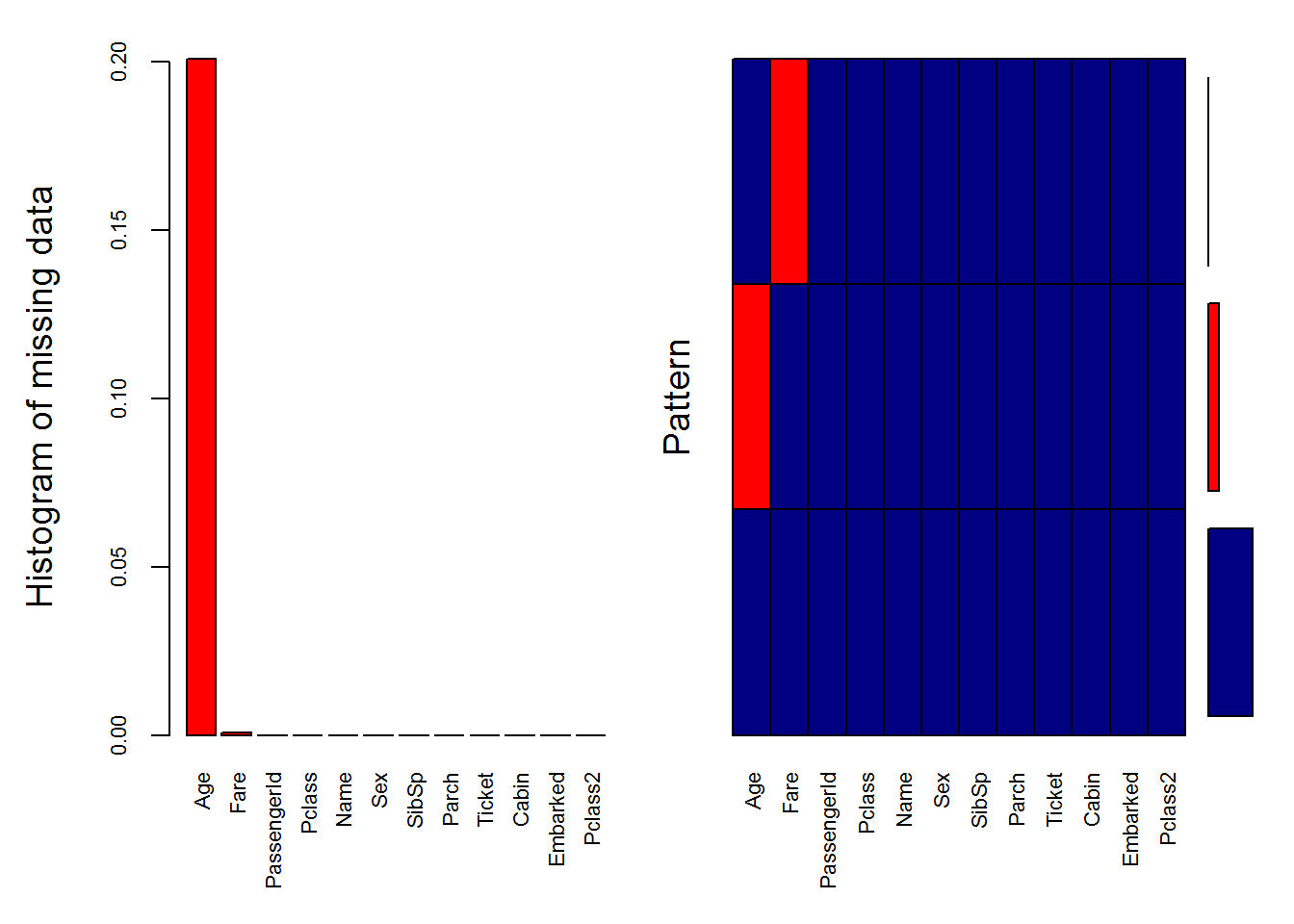## ## Variables sorted by number of missings: ## Variable Count ## Age 0.2009167303 ## Fare 0.0007639419 ## PassengerId 0.0000000000 ## Pclass 0.0000000000 ## Name 0.0000000000 ## Sex 0.0000000000 ## SibSp 0.0000000000 ## Parch 0.0000000000 ## Ticket 0.0000000000 ## Cabin 0.0000000000 ## Embarked 0.0000000000 ## Pclass2 0.0000000000 The histogram and resulting table indicate that roughly 20% of age values are missing, and that a very small percent (0.0007) of the fare values are missing.1 Logistic regression can handle missing values through listwise deletion, but this is not a sound strategy if we have the tools to impute missing values. Methods like Random Forests can’t work with missing values anyways, so we should really find a nice imputation solution. Let’s assume that these values are missing at random and are of an adequately low percentage of full data-frame representation.2 Since fare is only missing one, it is easily enough to impute using some central tendency measure. The mean is 33.29, while the median is 14.45. Inspecting the fare variable shows a number of problems. summary(comb_df$Fare)
##    Min. 1st Qu.  Median    Mean 3rd Qu.    Max.    NA's
##   0.000   7.896  14.450  33.300  31.280 512.300       1
par(mfrow=c(1,2))

plot(comb_df$Fare) hist(comb_df$Fare)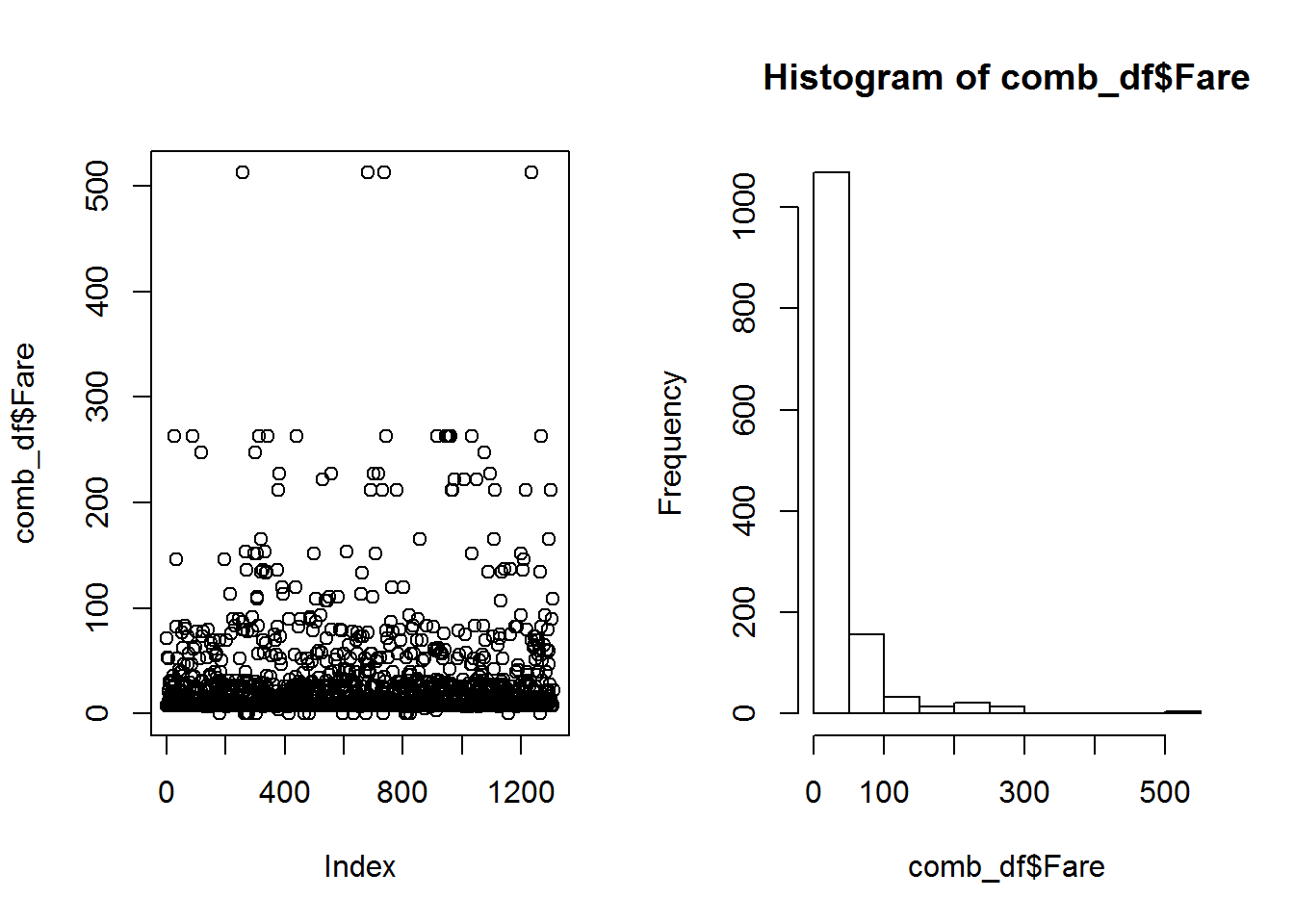First, there are Fares of 0. Did these people get on for free? Probably not. Second, it is clear upon inspection that outliers are pushing the mean Fare value up. Now we have more than just a simple imputation to deal with. We can impute the missing Fare with the median fare based on his class using the Rpart package, but first let’s set the zero values to NA and impute median values based on class.

comb_df$fare2 <- ifelse(comb_df$Fare==0, NA, comb_df$Fare) summary(comb_df$fare2) #inspect to see no more zero value, and now 18 NAs
##    Min. 1st Qu.  Median    Mean 3rd Qu.    Max.    NA's
##   3.171   7.925  14.500  33.730  31.330 512.300      18
library(rpart)

fareimp <- rpart(fare2 ~ Pclass, data=comb_df[!is.na(comb_df$fare2),], method="anova") comb_df$fare2[is.na(comb_df$fare2)] <- predict(fareimp, comb_df[is.na(comb_df$fare2),])

summary(comb_df$fare2) #inspect to see no more NAs ## Min. 1st Qu. Median Mean 3rd Qu. Max. ## 3.171 7.925 14.500 33.880 31.390 512.300 Now let’s impute age based on multiple covariates. ageimp <- rpart(Age ~ Pclass2 + Sex + SibSp + Parch + fare2 + Embarked, data=comb_df[!is.na(comb_df$Age),],
method="anova")

comb_df$age2 <- NA comb_df$age2[is.na(comb_df$age2)] <- predict(ageimp, comb_df[is.na(comb_df$age2),])

summary(comb_df$age2) #inspect to make sure. ## Min. 1st Qu. Median Mean 3rd Qu. Max. ## 9.60 27.43 27.43 29.63 32.10 40.55 Finally in terms of data extraction, let’s look at titles housed in the name variable. People more clever than I noticed that titles were consistent throughout the names listed in the passenger data. Historically, titles were also associated with different personal characteristics such as gender, class, nobility, etc. comb_df$Name <- as.character(comb_df$Name) strsplit(comb_df$Name, split='[,.]') #want to isolate the "title" string segment
## []
##  "Cumings"
##  " Mrs"
##  " John Bradley (Florence Briggs Thayer)"
comb_df$Title <- sapply(comb_df$Name, FUN=function(x) {strsplit(x, split='[,.]')[]})
comb_df$Title <- sub(' ', '', comb_df$Title)
comb_df$Title[comb_df$Title %in% c('Mme', 'Mlle')] <- 'Mlle'
comb_df$Title[comb_df$Title %in% c('Capt', 'Don', 'Major', 'Sir')] <- 'Sir'
comb_df$Title[comb_df$Title %in% c('Dona', 'Lady', 'the Countess', 'Jonkheer')] <- 'Lady'
comb_df$Title <- factor(comb_df$Title)
table(comb_df$Title) ## ## Col Dr Lady Master Miss Mlle Mr Mrs Ms Rev ## 4 8 4 61 260 3 757 197 2 8 ## Sir ## 5 Next, we can create a couple of family related variables. These are family size and family id or cluster: #family size. comb_df$FamilySize <- comb_df$SibSp + comb_df$Parch + 1 #add one to account for the passenger as well.

#combine surname and family size into a factor
comb_df$Surname <- sapply(comb_df$Name, FUN=function(x) {strsplit(x, split='[,.]')[]})
comb_df$FamilyID <- paste(as.character(comb_df$FamilySize), comb_df$Surname, sep="") comb_df$FamilyID[comb_df$FamilySize <= 2] <- 'Small' famIDs <- data.frame(table(comb_df$FamilyID))
famIDs <- famIDs[famIDs$Freq <= 2,] comb_df$FamilyID[comb_df$FamilyID %in% famIDs$Var1] <- 'Small'
comb_df$FamilyID <- factor(comb_df$FamilyID)
table(comb_df$FamilyID) ## ## 11Sage 3Abbott 3Boulos 3Bourke 3Brown ## 11 3 3 3 4 ## 3Caldwell 3Collyer 3Compton 3Coutts 3Crosby ## 3 3 3 3 3 ## 3Danbom 3Davies 3Dodge 3Drew 3Elias ## 3 5 3 3 3 ## 3Goldsmith 3Hart 3Hickman 3Johnson 3Klasen ## 3 3 3 3 3 ## 3Mallet 3McCoy 3Moubarek 3Nakid 3Navratil ## 3 3 3 3 3 ## 3Peacock 3Peter 3Quick 3Rosblom 3Samaan ## 3 3 3 3 3 ## 3Sandstrom 3Spedden 3Taussig 3Thayer 3Touma ## 3 3 3 3 3 ## 3van Billiard 3Van Impe 3Wells 3Wick 3Widener ## 3 3 3 3 3 ## 4Allison 4Baclini 4Becker 4Carter 4Dean ## 4 4 4 4 4 ## 4Herman 4Johnston 4Laroche 4West 5Ford ## 4 4 4 4 5 ## 5Lefebre 5Palsson 5Ryerson 6Fortune 6Panula ## 5 5 5 6 6 ## 6Rice 6Skoog 7Andersson 7Asplund 8Goodwin ## 6 6 9 7 8 ## Small ## 1074 Finally, let’s make a women and children first variable. This is based on the common anecdotal story of life boats only accepting women and children before adult males. comb_df$priority <- 0
comb_df$priority[which(comb_df$Sex=="female" | comb_df$age2 < 16)] <- 1 We have done a lot of work here! Let’s run some models. First resplit the combined data set back into training and test sets. train <- comb_df[1:891,] test <- comb_df[892:1309,] ## Logistic Regression Logistic regression is a classic classifier in statistics and econometrics. It is also heavily used in political science and sociology as a workhorse modeling method. Like linear regression and classification methods, we are interested in understanding the probability of an outcome $$Y=1$$ occurring conditional on a set of $$n$$ predictor variables within $$x\prime$$. $Pr(Y = 1|x\prime)$ One can read the above as being the probability of $$Y$$ equaling 1 given the measures found in $$x\prime$$. To model this predictive relationship, we can utilize conventions from linear regression. First we can represent the above conditional probability as: $Pr(Y = 1|x\prime) = p(x\prime)$ Next, propose a linear function of this probability: $p(x\prime) = \beta_{0} + \beta_{i}x_{i}...+ \beta_{n}x_{n}$ Unfortunately, stopping here would not help us. Our model would sometimes result in probabilities that are $$p(x\prime) < 0$$ or $$p(x\prime) > 1$$. It is impossible to have probabilities outside of the range $$p \in [0,1]$$. Fortunately, we can use the logistic function to guarantee outputs between 0 and 1 as such: $p(x\prime) = \frac{e^{\beta_{0}+\beta_{i}x_{i}...+\beta_{n}x_{n}}}{1 + e^{\beta_{0}+\beta_{i}x_{i}...+\beta_{n}x_{n}}}$ From here we can calculate the odds of $$p(x\prime$$): $\frac{p(x\prime)}{1 - p(x\prime)} = e^{\beta_{0} + \beta_{i}x_{i}...+\beta{n}x_{n}}$ Reiterating basic probability, the odds tells us about the probability of $$Y = 1$$. Odds close to 0 indicate very low probabilities for $$Y = 1$$, while probabilities ever approaching $$\infty$$ indicate very high probabilities for $$Y = 1$$. By logging both sides of the above odds equation, we can calculate the logit or logged odds: $log \bigg( \, \frac{p(x\prime)}{1 - p(x\prime)} \bigg) \, = \beta_{0} + \beta_{i}x_{i}...+ \beta{n}x_{n}$ This is the basis for using logistic regression to make classification predictions. We can interpret the influence of predictor $$x_{i}$$ as the change in the logged odds of $$Y = 1$$ by $$\beta_{i}$$. This is not a linear increase like the linear regression interpretation, but is a conditional increase based on the current value of $$x_{i}$$. Even though the relationship is non-linear, we can generalize the interpretation of $$\beta_{i}$$. If $$\beta_{i}$$ is positive, then increasing the value of $$x_{i}$$ will increase the logged odds of $$Y = 1$$. As such, a negative $$\beta_{i}$$ would have the opposite effect. The above seems easy enough, but how can we know the values of $$\beta_{0}$$, $$\beta_{i}$$, etc..? These parameters are generally estimated though the maximum likelihood method. Let’s assume $$n$$ observations and that $$x$$ and $$\beta$$ are sets of covariates and their corresponding beta estimates. First start with the likelihood function: $\ell(\beta_{0},\beta) = \prod_{i = 1}^{n}p(x_{i})^{y_{i}}(1 - p(x_{i}))^{1 - y_{i}}$ Through further derivation of the log-likelihood function, products turn into sums: $\ell(\beta_{0}, \beta) = \sum_{i=1}^{n}-log1 + e^{\beta_{0} + \beta x_{i}} + \sum_{i=1}^{n}y_{i}(\beta_{0} + \beta x_{i})$ Finally, one can find the maximum likelihood estimates for a specific parameter by taking the partial derivative of the log likelihood function. Say we wanted the estimate $$\beta_{j}$$ from the set of estimates $$\beta$$. We take the partial derviative of the log likelihood function with respect to $$\beta_{j}$$ $\frac{\partial \ell}{\partial \beta_{j}} = -\sum_{i=1}^{n} \frac{1}{1 + e^{\beta_{0} + \beta x_{i}}} e^{\beta_{0} + \beta x_{i}}x_{ij} + \sum_{i=1}^{n} y_{i}x_{ij}$ $= \sum_{i=1}^{n}(y_{i} - p(x_{i};\beta_{0},\beta))x_{ij}$ From here, we have the basis for estimating $$\beta_{j}$$. The resulting beta values are estimates because the partial derivative function is transcendental. ### Logistic Regression using GLM So let’s put the above into action. Logitistic regression models are easy to implement in R using the glm() function. Here I use the logit link. lfit <- glm(Survived2 ~ Pclass2 + SibSp + fare2 + Embarked + priority + age2 + Parch + Title, data = train, family = binomial(logit)) summary(lfit) ## ## Call: ## glm(formula = Survived2 ~ Pclass2 + SibSp + fare2 + Embarked + ## priority + age2 + Parch + Title, family = binomial(logit), ## data = train) ## ## Deviance Residuals: ## Min 1Q Median 3Q Max ## -2.4405 -0.6336 -0.3982 0.5188 2.4633 ## ## Coefficients: ## Estimate Std. Error z value Pr(>|z|) ## (Intercept) 1.114166 1.749083 0.637 0.52412 ## Pclass22 -1.086626 0.403463 -2.693 0.00708 ** ## Pclass23 -2.181913 0.444036 -4.914 0.000000893 *** ## SibSp -0.438038 0.143767 -3.047 0.00231 ** ## fare2 0.002477 0.002457 1.008 0.31342 ## EmbarkedQ -0.108594 0.395787 -0.274 0.78380 ## EmbarkedS -0.456701 0.245963 -1.857 0.06334 . ## priority -2.171446 0.979025 -2.218 0.02656 * ## age2 -0.023742 0.024821 -0.957 0.33880 ## Parch -0.360929 0.136907 -2.636 0.00838 ** ## TitleDr 0.467218 1.652747 0.283 0.77741 ## TitleLady 2.478722 2.116785 1.171 0.24160 ## TitleMaster 4.471005 1.645752 2.717 0.00659 ** ## TitleMiss 4.874589 1.739323 2.803 0.00507 ** ## TitleMlle 17.430855 840.228654 0.021 0.98345 ## TitleMr -0.338500 1.440880 -0.235 0.81427 ## TitleMrs 5.381037 1.756308 3.064 0.00219 ** ## TitleMs 18.896646 1455.398594 0.013 0.98964 ## TitleRev -14.406445 591.633321 -0.024 0.98057 ## TitleSir -0.156936 1.700415 -0.092 0.92647 ## --- ## Signif. codes: 0 '***' 0.001 '**' 0.01 '*' 0.05 '.' 0.1 ' ' 1 ## ## (Dispersion parameter for binomial family taken to be 1) ## ## Null deviance: 1186.66 on 890 degrees of freedom ## Residual deviance: 729.89 on 871 degrees of freedom ## AIC: 769.89 ## ## Number of Fisher Scoring iterations: 14 dev = 1186.6 - 630.75 dfree <- 890 - 812 sig <- 1 - pchisq(dev, df=dfree) sig ##  0 #significant model at the 0% level anova(lfit, test="Chisq") ## Analysis of Deviance Table ## ## Model: binomial, link: logit ## ## Response: Survived2 ## ## Terms added sequentially (first to last) ## ## ## Df Deviance Resid. Df Resid. Dev Pr(>Chi) ## NULL 890 1186.66 ## Pclass2 2 103.547 888 1083.11 < 0.00000000000000022 *** ## SibSp 1 0.040 887 1083.07 0.8417158 ## fare2 1 7.479 886 1075.59 0.0062416 ** ## Embarked 2 16.721 884 1058.87 0.0002339 *** ## priority 1 238.582 883 820.29 < 0.00000000000000022 *** ## age2 1 3.652 882 816.63 0.0559969 . ## Parch 1 0.941 881 815.69 0.3320660 ## Title 10 85.802 871 729.89 0.00000000000003626 *** ## --- ## Signif. codes: 0 '***' 0.001 '**' 0.01 '*' 0.05 '.' 0.1 ' ' 1 vif(lfit) ## GVIF Df GVIF^(1/(2*Df)) ## Pclass2 4.533083 2 1.459145 ## SibSp 2.085296 1 1.444055 ## fare2 1.640160 1 1.280687 ## Embarked 1.309050 2 1.069644 ## priority 27.055227 1 5.201464 ## age2 4.978660 1 2.231291 ## Parch 1.521050 1 1.233309 ## Title 46.902284 10 1.212159 test$Survived <- predict(lfit, newdata = test, type="response")
test$Survived[test$Survived > .5]="Yes"
test$Survived[test$Survived <= .5]="No"
vars <- c("PassengerId", "Survived")
sub <- test[vars]
sub$Survived[sub$Survived=="Yes"]=1
sub$Survived[sub$Survived=="No"]=0
sub$Survived <- as.factor(sub$Survived)
write.csv(sub, "besaw_sub.csv")

Score of 0.79426, not bad…Let’s try some interaction effects.

lfit <- glm(Survived2 ~ Pclass2 + SibSp + fare2 + Embarked + priority + age2 + Parch + Title + priority*Pclass2 +
SibSp*priority + Pclass2*SibSp + Pclass2*Parch,
data = train, family = binomial(logit))

summary(lfit)
##
## Call:
## glm(formula = Survived2 ~ Pclass2 + SibSp + fare2 + Embarked +
##     priority + age2 + Parch + Title + priority * Pclass2 + SibSp *
##     priority + Pclass2 * SibSp + Pclass2 * Parch, family = binomial(logit),
##     data = train)
##
## Deviance Residuals:
##     Min       1Q   Median       3Q      Max
## -3.0165  -0.5355  -0.4199   0.3414   2.3268
##
## Coefficients:
##                      Estimate  Std. Error z value Pr(>|z|)
## (Intercept)          0.679764    1.914146   0.355  0.72249
## Pclass22            -1.604792    0.547557  -2.931  0.00338 **
## Pclass23            -1.345472    0.536659  -2.507  0.01217 *
## SibSp                0.305911    0.357008   0.857  0.39151
## fare2                0.001726    0.002698   0.640  0.52227
## EmbarkedQ           -0.155566    0.381959  -0.407  0.68380
## EmbarkedS           -0.535461    0.250159  -2.140  0.03232 *
## priority            -0.140686    1.170246  -0.120  0.90431
## age2                -0.011483    0.031468  -0.365  0.71519
## Parch               -0.744546    0.418493  -1.779  0.07522 .
## TitleDr             -0.097666    1.689988  -0.058  0.95392
## TitleLady            0.816524    2.033841   0.401  0.68808
## TitleMaster          4.284450    1.700119   2.520  0.01173 *
## TitleMiss            4.415994    1.798349   2.456  0.01407 *
## TitleMlle           15.384437  840.259572   0.018  0.98539
## TitleMr             -0.600261    1.449138  -0.414  0.67871
## TitleMrs             4.603745    1.813619   2.538  0.01114 *
## TitleMs             17.652788 1455.398737   0.012  0.99032
## TitleRev           -13.915157  589.976359  -0.024  0.98118
## TitleSir            -0.362893    1.712217  -0.212  0.83215
## Pclass22:priority   -0.139366    0.974519  -0.143  0.88628
## Pclass23:priority   -2.485059    0.842521  -2.950  0.00318 **
## SibSp:priority      -0.321285    0.382498  -0.840  0.40093
## Pclass22:SibSp      -0.559350    0.606727  -0.922  0.35657
## Pclass23:SibSp      -0.469754    0.476165  -0.987  0.32387
## Pclass22:Parch       1.193951    0.607597   1.965  0.04941 *
## Pclass23:Parch       0.424308    0.443516   0.957  0.33872
## ---
## Signif. codes:  0 '***' 0.001 '**' 0.01 '*' 0.05 '.' 0.1 ' ' 1
##
## (Dispersion parameter for binomial family taken to be 1)
##
##     Null deviance: 1186.66  on 890  degrees of freedom
## Residual deviance:  691.46  on 864  degrees of freedom
## AIC: 745.46
##
## Number of Fisher Scoring iterations: 14

Deviance change seemed promising, but a score of 0.78, not an improvement…

1. It is actually just one..

2. Multiple imputation assumes that missing values are missing at random (MAR). One should be careful when performing multiple imputation on real world data, as the MAR assumption is often not feasible. I am not sure why Age has so many missing values here. It could be a design by Kaggle, which is what I personally assume for this analysis. Normally, if one variable has such a high degree of missingness, it is unlikely to be missing at random. In addition, missing values should normally not be more than 5%-10% of a measure’s representation. Having much higher degrees of missingness is often a sign of MAR violation. Consequently, with more missingness, you are more likely to artificially construct patterns within the imputed data if your baseline data is unrepresentative of the real population.

# Test Python Code

Here is some python code for making a simple number guessing game.

import random

guessesTaken = 0

my_name = input("Name: ", )

number = random.randint(1, 20)
print("Well, " + my_name + ", I am thinking  of a number between 1 and 20.")

while guessesTaken < 6:
print("Take a guess.")
guess = int(input())

guessesTaken = guessesTaken + 1

if guess  < number:

if guess > number:

if guess == number:
break

if guess == number:
guessesTaken = str(guessesTaken)
print("Good job, " + my_name + "! You guessed my number in " + guessesTaken + ' guesses!')

if guess != number:
number = str(number)
print("Nope. The number I was thinking of  was " + number)



# Intro to Bayesian methods

This post is really only a test for Rmarkdown integration into Jekyll. Substantively, it is a first lab lesson for applied Bayesian estimation in R.

Load the LearnBayes1 and Lattice packages.

1. Please replicate the sleeping habits analysis using a discrete prior (in the session 1-2)

Setting up a discrete prior

This code is used to create a a discrete prior concerning our beliefs about the proportion college student sleeping habits (p):

The code above is two-fold, first, it sets up the range of possible proportion values for the amount of students that could be sleeping at least eight hours a night. Second, it creates our discrete prior by specifying our weighted beliefs about each distinct proportion (p).

Next, we plot the our priors for each proportion (p) by using the lot function: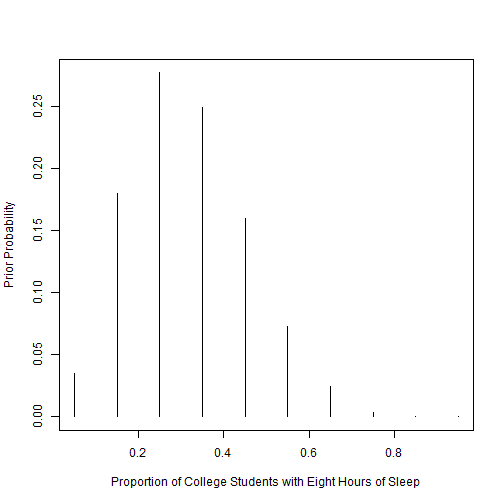The histogram above visualizes our prior belief in each value of p. The original guess of .3 (from lesson 1-2) being the most probable is highlighted clearly in the histogram. We see that our prior beliefs are greatest between .2 and .4, with.3 being the peak in the middle.

Evidence and Posterior

Next, we want to calculate the posterior probabilities for p by utilizing the data on college student sleeping habits. In the example study, only 11 out of 27 participants were found to have slept a sufficient number of hours per night. By using the pdisc function from the LearnBayes package, we can utilize our established priors to compute our posterior probabilities. The code below is used to create our posterior probabilities:

First, the code above establishes our evidence (data) from the example study (11 students out of 27). Second, the posterior values are calculated using the pdisc function, which takes our discrete values (p), our priors, and the evidence. The function below provides simple table of the work conducted so far:

Here we can easily see the prior and posterior probabilies for each level of p.

Comparsion of Prior and Posterior

By using the lattice package, and the xyplot function, R can visualize the comparison between the prior and posterior probabilities. The code below sets up the comparison graphs:

The above code creates a dataset composed of the prior and posterior distributions. Next, this dataset is used to create a set of comparison graphs for the priors and the posteriors: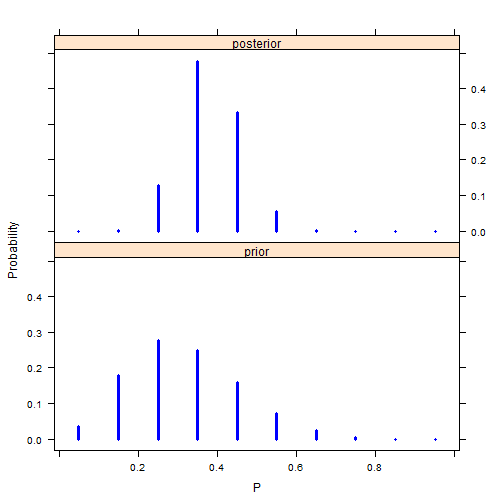Here we can see a direct visual comparison between the prior and posterior probability distributions. Taking the evidence into account, we see that posterior probability peak shifts closer to .4. Overall, the main set of probability distributions is settled in-between .25 and .45 in terms of proportion (p).

2. Please replicate the sleeping habits analysis using a beta prior (in the session 1-2b).

Setting up the Beta Prior

The specification of a beta distribution is useful for bayesian analysis when it concerns the behavior of percentages and proportions.2 The beta distribution is reflected by two shape parameters a and b, which can represent our prior beliefs about the proportion of students who get at least eight hours of sleep a night p. The code below creates the shape parameters for the beta prior by using the beta.select function, which utilizes our examples prior belief about two quantiles of p, the median (.3) and and 90th percentile (.5).3 Our example study believes that the median is equally likely (.5) to be smaller or larger than the median (.3), and that p is less than .5 (90th Percentile), with 90% confidence.

The beta.select function provides us with the shape parameters of a(3.26) and b(7.19). This was done by first creating two variables that lists our beliefs about the median (.3) and 90th percentile (.5) quantiles, and using them as arguments in the beta.select function.

Evidence and Posterior

For the evidence and likelihood function, we retain the previous example study data of 11 college students out of 27 who receive at least eight hours of sleep. A conjugate analysis is possible with the beta prior, because once you combine the beta prior with the evidence, a beta posterior is computed. The code below sets up the beta posterior by using the dbeta command: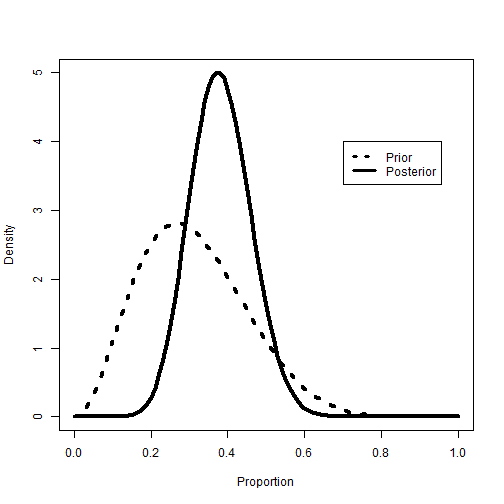Here we can see a visualized comparison between the beta prior and posterior. As with the discrete posterior, the peak probability density for the beta posterior shifts towards .4 for p, after we take the study evidence into account.

Analyzing the beta posterior

This section looks at three different ways in which the beta posterior can be analyzed. For this, we ask the question; Is it likely that the proportion of heavy sleepers is greater than .5?

1) The first way to analyze this question is to compute the probability of p being greater than .5 by using the pbeta function, which is the beta distribution function. The code below calculates the probability of our question being right:

The probability calculated is 6.9%, which is small. We can be confident that p is probably not more than .5, or more than half of college student sleepers.

2) The seoncd way to analyze this question is to create a credible interval estimate, which is a bayesian take on frequentist confidence intervals.4 Credible Intervals can be calculated by using the qbeta function in R. The code below calculates the credible intervals for the beta posterior:

The calculated 90% credible interval for our beta posterior is (0.256, 0.513). Again, it seems unlikely that p is greater than .5 because the credible interval only reaches past .5 on the uppertail, with the majority of the interval being below .5.

3) The third way to analyze this question is to use a simulation of a large number of values from the beta posterior. To do this, the rbeta function is used to generate a large amount of random proportion values based on the beta shape paramters. The code below computes the simulation and visualizes the results using the hist function: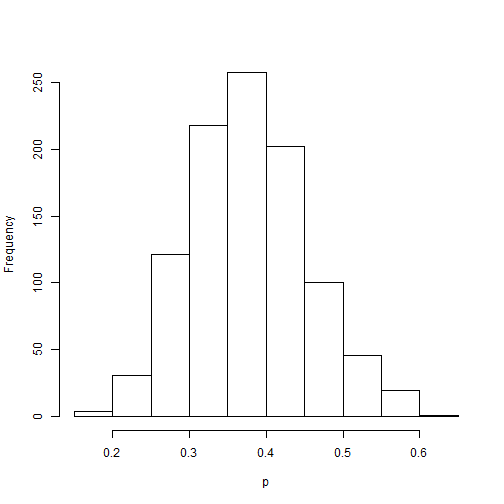The histogram above shows newly simulated sample for p. Next we can replicate the previous two posterior analyzes by using the sum and quantile functions. The code below calculates the probability and credible interval for the question of interest:

Both the probability (6.9% vis a vis 6.7%) and the credible interval estimates (0.25, 0.51 vis a vis .26, .52) are very close to the original exact values of the beta posterior. The results still lead to the analyst to conclude that p is unlikely to be greater than .5, but what if we can increase percision by greater simulation? The next block of code replicates the previous simulation procedure but with 1,000,000 generated values for p instead.

After increasing the simulation to a 1,000,000 values of p, we find even closer estimates of the probability of p > .5 (6.9% vis a vis 6.8%) and the credible interval (0.25, 0.51 vis a vis .25, 0.51). We can be fairly confident in our previous findings concerning the question. After taking into account the study evidence, it is unlikely that the proportion of college students who have at least eight hours of sleep a night is greater than .5.

3. Please replicate the birth rate comparison analysis using a Poisson Likelihood (in the session 1-5).

For the study of birth rates amongst women with, and without college degrees, we utilize the Poisson model. Birthrates qualify as count data, which can be better analyzed by using poisson and negative binomial modeling.

Setting up the Prior

For a Poisson likelihood model, one can use a gamma conjugate prior. Again, this type of prior only needs to model our beliefs about two parameters, a(occurances) and b(intervals). The code below sets up our gamma prior.

Evidence and Posterior

Next, we wish to take into account the evidence about birthrates amongst women without college degrees, and with college degrees. This data comes from the General Social Survey (GSS), which interviewed women on educational attainment and number of children. From here, group 1 defines women without a college degree, and group 2 defines women with a college degree. The code below establishes the evidence (data) for both groups of women, and then finds the 95% credible interval for number of children for each group.

The posterior mean number of children born to group 1 is 1.95, the mode is 1.94, and the 95% credible interval is (1.70, 2.22). The code below computes the posterior data for group 2.

The posterior mean number of children born to group 2 is 1.51, the mode is 1.48, and the 95% credible interval is (1.17, 1.89).

Posterior Group Comparison

Comparing the posterior results between group 1 (without degrees) and group 2 (with degrees) shows that women with degrees appear to have less children (y) on average than their counterparts without degrees (1.51 versus 1.95). The posterior credible intervals also show two mostly different ranges for number of children (y) had by both groups of women (1.70, 2.22 versus 1.17, 1.89). The code below creates a visualized comparison of the gamma prior, posterior for group 1, and posterior for group 2: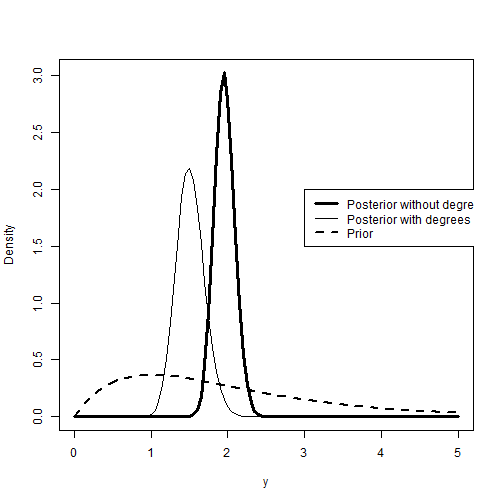The plot above confirms the previous observation about the posterior comparison between the two groups of women. Women without degrees peaks around 2 children, while women with degrees peaks at around 1.5 children.

Birth Rate Prediction

To make predictions about the number of children women will have depending on their college degree status, we can utilize the posteriors found above with a negative binomial distribution. This can be done in r by using the dnbinom function. The code below creates predictions of birth rates (y) for both groups of women:

The code above creates predicted probabilities for number of children born on an interval of 0 to 10. The dnbinom function uses two arguments to create the predicted probabilities. The first is the size, or dispersion paramters, and the second argument is the mean.

The code below creates a visualized comparison of the predicted probabilities for both groups of women: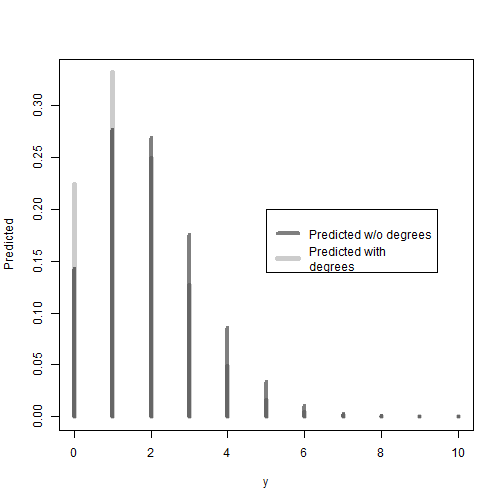As expected, the plot above shows that women without degrees have higher predicted probabilities to have two or more children. In turn, women with a degree have relatively higher predicted probabilities for having a single child or no child, when compared to women without a degree.

1. LearnBayes is a package created by Jim Albert at Bowling Green University. Documentation can be found at http://cran.r-project.org/web/packages/LearnBayes/LearnBayes.pdf.

2. Kruschke, John. Doing Bayesian data analysis: A tutorial introduction with R. Academic Press, 2010.

3. Beta priors can also be established thorugh the use of our beliefs concerning the beta distribution’s mean and standard deviation, but this can be problematic, because the analyst must make sure the standard deviation makes sense for a beta distribution (Kruschke, J, 2010).

4. Credible Intervals are essentially analagous to Confidence Intervals in frequentist statistical analysis, but differ in a key way. Credible intervals incorporate contextual information from the prior, while confidence intervals take only the data (evidence) into account. For more on credible intervals vis a vis confidence intervals, please see: Greenberg, Edward. Introduction to Bayesian econometrics. Cambridge University Press, 2012.

# Site launch!

I have finally figured out Github page hosting with Jekyll. I would like to thank Barry Clark for his incredible walkthrough for using Jekyll Now as a static site generator. Additionally, I would like to acknowledge Mark Otto as the author/creator of the Lanyon theme for Jekyll that is used by this site.